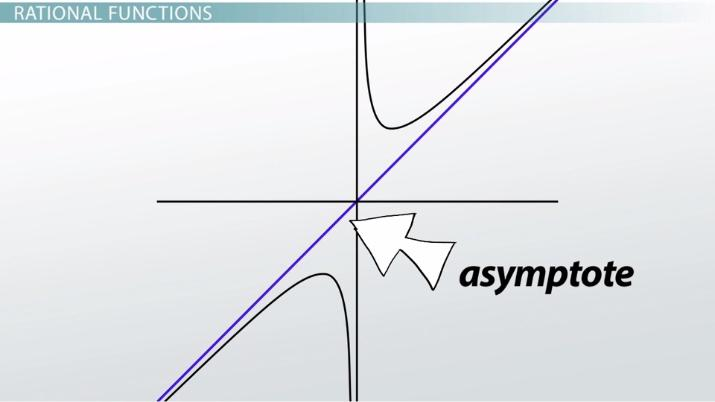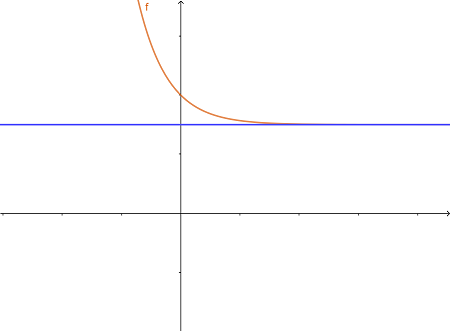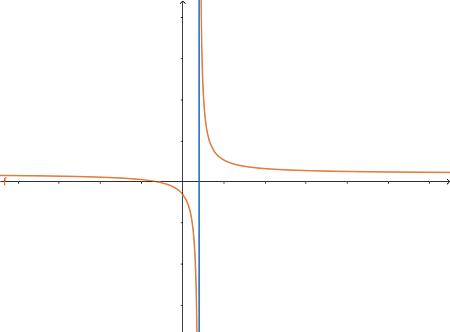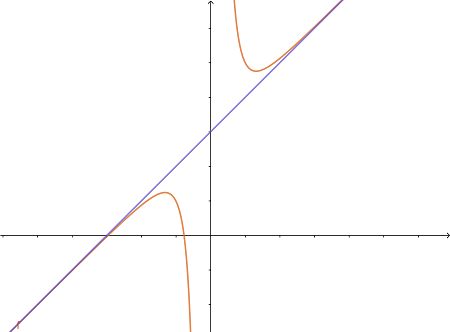# Finding Slant Asymptotes of Rational Functions

Noah Kaufmann, Yuanxin (Amy) Yang Alcocer
• Author
Noah Kaufmann

Noah is a fifth year graduate student in the math department at Indiana University. He also has a B.S. and M.S. from The University of Iowa, and has been tutoring students in math for almost ten years.

• Instructor
Yuanxin (Amy) Yang Alcocer

Amy has a master's degree in secondary education and has been teaching math for over 9 years. Amy has worked with students at all levels from those with special needs to those that are gifted.

Learn about slant asymptotes, what they look like, and the rules to calculate them. Understand how to find a slant asymptote with different examples provided. Updated: 01/15/2022

Show

## Slant Asymptote

A slant (also called oblique) asymptote for a function {eq}f(x) {/eq} is a linear function {eq}g(x) {/eq} with the property that the limit as {eq}x {/eq} approaches {eq}\pm\infty {/eq} of {eq}f(x) {/eq} is equal to {eq}g(x) {/eq}. In this lesson, we will see how to find slant asymptotes using either long division or synthetic division.

### Horizontal, Vertical & Slant Asymptote Graph

There are two other types of asymptotes that usually are taught before slant asymptotes: vertical and horizontal. A vertical asymptote appears whenever a function approaches either positive or negative infinity at a point, and horizontal asymptotes occur when a function has a constant limit at either positive or negative infinity. Slant asymptotes occur when the limit of a function at positive or negative infinity is a linear function. Let's see some examples of what each of these look like graphically:An error occurred trying to load this video.

Try refreshing the page, or contact customer support.

Coming up next: Expressions of Rational Functions

### You're on a roll. Keep up the good work!

Replay
Your next lesson will play in 10 seconds
• 0:02 Rational Functions
• 2:41 Finding the Slant Asymptote
• 3:56 Example 1
• 5:05 Example 2
• 6:34 Lesson Summary
Save Save

Want to watch this again later?

Timeline
Autoplay
Autoplay
Speed Speed## Slant Asymptote Rules

Vertical and horizontal asymptotes can occur in a variety of different types of functions. However, slant asymptotes most commonly appear for two types of functions. The first type of function is a rational function, of the form {eq}\frac{f(x)}{g(x)} {/eq}, where {eq}f(x) {/eq} and {eq}g(x) {/eq} are both polynomials, and the degree of {eq}f(x) {/eq} must be exactly one higher than the degree of {eq}g(x) {/eq}. The second type of function is an irrational function (a function containing roots) where each root contains a polynomial of degree equal to the power of that root. That might sound a bit confusing, but it just means that if there is a square root it needs to contain a quadratic, a cube root should contain a cubic function, and so on.

Let's look at an example. Suppose we have the function {eq}\sqrt{x^2+x+1} {/eq}. Since this is a quadratic polynomial inside a square root, it will have a slant asymptote. To find the equation of this slant asymptote, we will use the definition of slant asymptote and properties of limits.

First, since a slant asymptote is a linear function, we know it must be equal to {eq}ax+b {/eq}. The formal definition of a slant asymptote is that {eq}\lim_{x\to\pm\infty} f(x) - (ax+b) = 0 {/eq}. Dividing both sides by {eq}x {/eq} gives us that {eq}\lim_{x\to\pm\infty} \frac{f(x)}{x} - a - \frac{b}{x} = 0 {/eq}. Since we're taking the limit at {eq}\pm\infty {/eq}, the {eq}-\frac{b}{x} {/eq} term disappears, and we get {eq}\lim_{x\to\pm\infty} \frac{f(x)}{x} - a = 0 {/eq}. So then {eq}\lim_{x\to\pm\infty} \frac{f(x)}{x} = a {/eq}, and now we have a method for finding {eq}a {/eq}.

Let's apply this method to our function to find {eq}a {/eq}, and then we will explain the method for finding {eq}b {/eq}.

We proceed as follows: {eq}\lim_{x\to\pm\infty} \frac{\sqrt{x^2+x+1}}{x} = \lim_{x\to\pm\infty} \frac{\sqrt{x^2(1+\frac{1}{x}+\frac{1}{x^2})}}{x} = \lim_{x\to\pm\infty} \frac{x\sqrt{(1+\frac{1}{x}+\frac{1}{x^2})}}{x} = \lim_{x\to\pm\infty} \sqrt{(1+\frac{1}{x}+\frac{1}{x^2})} = \lim_{x\to\pm\infty} \sqrt{(1)} = 1 {/eq}

Therefore {eq}a = 1 {/eq}, and our slant asymptote has the form {eq}x + b {/eq}. To solve for {eq}b {/eq} we can simply go back to the definition of slant asymptote, which is that {eq}\lim_{x\to\pm\infty} f(x) - (ax+b) = 0 {/eq}. If we add {eq}b {/eq} to both sides of this equation, we have a method for finding {eq}b {/eq} once we know {eq}a {/eq}: {eq}\lim_{x\to\pm\infty} f(x) -ax = b {/eq}.

Now let's apply this method to our current problem.

{eq}\lim_{x\to\pm\infty} \sqrt{x^2+x+1} -x = \lim_{x\to\pm\infty} (\sqrt{x^2+x+1} -x) (\frac{\sqrt{x^2+x+1} +x}{\sqrt{x^2+x+1} +x}) = \lim_{x\to\pm\infty} \frac{x+1}{\sqrt{x^2+x+1} +x} = \lim_{x\to\pm\infty} \frac{1+\frac{1}{x}}{\sqrt{1+\frac{1}{x}+\frac{1}{x^2}} +1} = \lim_{x\to\pm\infty} \frac{1}{\sqrt{1}+1} = \frac{1}{2} = b {/eq}

Therefore the slant asymptote for {eq}\sqrt{x^2+x+1} {/eq} is {eq}x+\frac{1}{2} {/eq}.

## How to Find the Slant Asymptotes?

The above method works for most types of functions with slant asymptotes, as long as the limits can be evaluated. However, for rational functions with slant asymptotes, like {eq}\frac{x^3 + 2x^2 + 3}{x^2 + 4} {/eq}, we can also use polynomial division to find their asymptotes. There are two main methods for doing this: long division and synthetic division. Polynomial long division is essentially the same as the standard long division for numbers, and synthetic division is a special form of long division that only works for linear factors. However, long division also works for linear factors, so if the method of synthetic division seems confusing, don't worry about it!

### Slant Asymptote Example 1

Let's briefly review polynomial long division and show how to find the slant asymptote of a function using this method. Using our function {eq}\frac{x^3 + 2x^2 + 3}{x^2 + 4} {/eq} from earlier, we place the numerator and denominator into the following positions:

{eq}\begin{align*} &\text{ }\text{ }\text{ }\\ x^2 + 0x + 4 &\overline{\big)x^3+2x^2+0x+3}\\ \end{align*} {/eq}

To unlock this lesson you must be a Study.com Member.

#### How do you find the slant asymptote?

The slant asymptote can be found using the limit method shown in the lesson, or for rational functions by using either polynomial long division or synthetic division.

#### What is the rule for oblique asymptotes?

A rational function will have a slant or oblique asymptote if the degree of the numerator is exactly one higher than the degree of the denominator.

#### Are slant and oblique asymptotes the same?

Slant asymptotes and oblique asymptotes are two different terms for the same thing: an asymptote which has a linear equation.

### Register to view this lesson

Are you a student or a teacher?